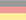# Elementary mathematics

Based on "A Calculus of Number Based on Spatial Forms" by Jeffrey M. James 1993.
```f1(x) = x
f2(x) = -x
f3(x) = ex
f4(x) = ln x
f3(f4(x)) = x   eln x = x
f4(f3(x)) = x   ln ex = x

<>     - Inversion        -0 = 0
() = o - Instanziation    e0 = 1
[] = ∎ - Abstraction    ln 0 = -∞ + [-∞...∞]i

[(a)] = ([a]) = a     Involution                                 Axiom 1     ln ea = eln a = a
(a[bc]) = (a[b])(a[c])  Distribution of a                          Axiom 2     ea + ln (b + c) = ea + ln b + ea + ln c
a<a> =               Inversion of a                             Axiom 3     a - a = 0
<<a>> = a             Inverse Cancellation  (double Inversion)   Theorem 1   -(-a) = a
<a><b> = <ab>          Inverse Collection                         Theorem 2   (-a) + (-b) = -(a + b)

Proof of Inverse Cancellation     for a != ∎
<<a>>
<<a>><a>a   -Inversion of a
a   Inversion of <a>

Proof of Inverse Collection     for a,b != ∎
<a><b>
<a><b>ab<ab>   -Inversion of ab
<ab>   Inversion of a and b

Proof of additive self-inversion of void (ambiguous sign)   -0 = 0
<>
Inversion of void

Cardinality of a (aggregation second degree)
a = ([a]) = ([a][o])             -Involution2, -Involution1
aa = ([a][o])([a][o]) = ([a][oo]) 2x a, -Distribution of [a]
a...a = ([a][o...o])                 induction step to all natural numbers

a * b    =>  ([a][b])
a / b    =>  ([a]<[b]>)     for b !=

Proof of Associativity of multiplication   (a*b)*c = a*(b*c)
([([a]  [b])][c]  )
(  [a]  [b]  [c]  )   Involution1
(  [a][([b]  [c])])   -Involution1

Proof of multiplicative self-inversion of o   1/1 = 1
(<[o]>)
(<   >)   Involution1
(     )   Inversion of void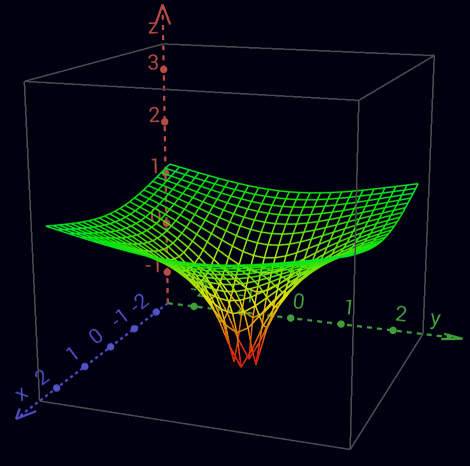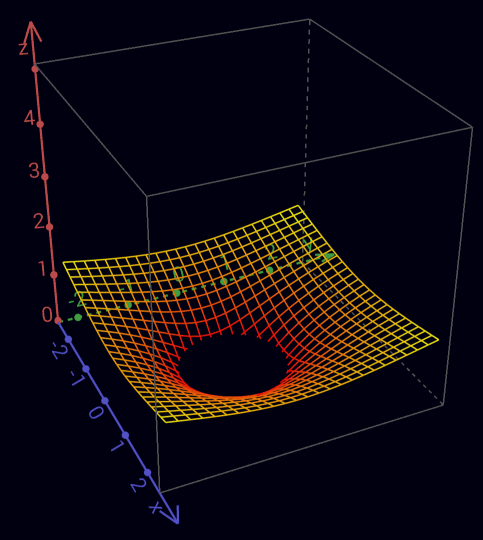Real part of the complex logarithm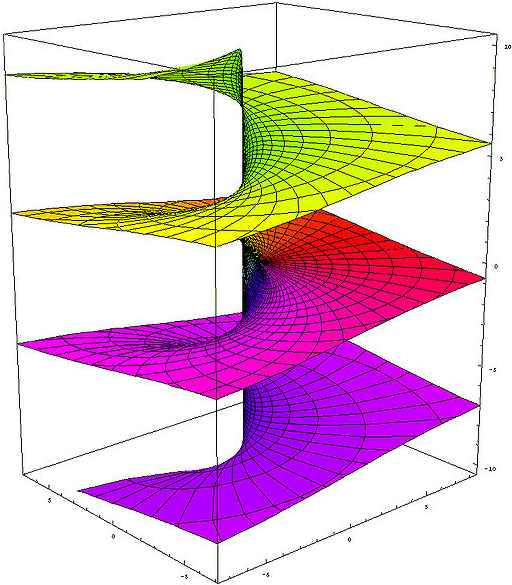Imaginary part of the complex logarithm - Riemann surface

Absorption
([a]∎) =      Zero cardinality of a             Theorem 3   a * 0 = 0
∎ a = ∎    Absorption of a (black hole)      Theorem 4   ∎ + a = ∎

Proof of Zero cardinality
([a]∎)
([a]∎)([a][b])<([a][b])>   -Inversion of ([a][b])
([a][b])<([a][b])>   -Distribution of [a]
Inversion of ([a][b])

Proof of Absorption
∎   a
[(∎ [(a)])]   2x -Involution1
[         ]   Zero cardinality of (a)

Proof of Zero cardinality
([a]∎)
(   ∎)   Absorption of [a]
Involution2

Indefiniteness of cardinality of ∎
∎ = ∎∎ = ∎∎∎ = ∎∎∎∎ = ...    -Absorption of ∎

= <> = [o] = (∎) = [(<>)] = ([<>]) =  <[o]> = <(∎)> 5x Inversion of void, 3x Involution1, 3x Involution2

Indefiniteness of Inversion of ∎
(<∎>) = ([o]<∎>) <= 1/0 is undefined (singularity)

0 * ? = 1   zero don't have multiplicative inverse
0/0 ambiguous
0/a = 0   a/a = 1   a/0 = ∞

inverse Absorption
<∎>  a
<∎><<a>>   -Inverse Cancellation
<∎  <a>>   Inverse Collection
<∎     >   Absorption of <a>

∎<∎>   -Inversion of ∎
=>  Absorption of <∎> or inverse Absorption of ∎
=>  Possibility of arbitrary absorption of everything everywhere
=>  <∎> undefined (Inversion of a black hole is not allowed)
=>  Division by 0 is undefined (singularity)

Cardinality of [a] (aggregation third degree)
([a]...[a]) = (([[a]][o...o]))
Since multiplication is as commutative as addition, factors can be aggregated just like summands.

Addition         2 + 4                 = 6
Multiplikation   2 * 4 = 2 + 2 + 2 + 2 = 8
Exponentiation   24    = 2 * 2 * 2 * 2 = 16
Tetration        42   = 2222 = 224 = 216 = 65.536
Hyper-5          24 = 2222 = 422 = 65.5362 = 2...2 65.536 times - therefore, our universe is far too small
...

Since exponentiation is not commutative, exponents cannot be represented aggregated like summands or factors.
Thus aggregations lose their beauty and thus their usefulness from here on.
oo     ooo                                   2 + 3             = 5
(  [oo]   [ooo] )            =   oo  oo  oo      2 * 3 = 2 + 2 + 2 = 6
(([[oo]]  [ooo]))            = ([oo][oo][oo])    23    = 2 * 2 * 2 = 8
(([[oo]][ (([[oo]][oo])) ])) = (([[oo]][oooo]))  32    = 222  = 24  = 16
(([[oo]][ (([[oo]][ (([[oo]][oo])) ])) ]))       23 = 222 = 42 = 2222 = 65.536
...

... = 22 = 22 = 22 = 2*2 = 2+2 = 4

ab      =>  (([[a]][b]))
a0 = 1  =>  (([[a]]∎)) = ((∎)) = o                  Absorption of [[a]], Involution2
a1 = a  =>  (([[a]][o])) = (([[a]])) = ([a]) = a    Involution1, 2x Involution2

Inversion
([<a>]b) = <([a]b)>        Inverse Promotion1      Theorem 5   -a * eb = -(a * eb)
(<[<a>]>) = <(<[a]>)>      Inverse Promotion2      Axiom 4     1/-a = -1/a
(<[<a>]>b) = <(<[a]>b)>    Inverse Promotion3      Theorem 6   1/-a * eb = -(1/a * eb)

Proof of Inverse Promotion1     for a != ∎
([<a>]b)
<([a]b)>([a]b)([<a>]b)    -Inversion of ([a]b)
<([a]b)>([a     <a>]b)    -Distribution von b
<([a]b)>([         ]b)    Inversion of a
<([a]b)>([         ] )    Absorption von b
<([a]b)>                  Involution2

Proof of Inverse Promotion3     for a != ∎
(   <[<a>]>   b)
<(<[a]>b)>(  <[a]>  b)(   <[<a>]>   b)    -Inversion of (<[a]>b)
<(<[a]>b)>([(<[a]>)]b)([ (<[<a>]>) ]b)    2x -Involution1
<(<[a]>b)>([(<[a]>)      (<[<a>]>) ]b)    -Distribution von b
<(<[a]>b)>([(<[a]>)     <(<[ a ]>)>]b)    Inverse Promotion2
<(<[a]>b)>([                       ]b)    Inversion of (<[a]>)
<(<[a]>b)>([                       ] )    Absorption von b
<(<[a]>b)>                                Involution2

Proof of irrationality of √2
√2  = p/q and  p, q ∈ ℤ shall be divisor extraneous
2   = (p/q)2
2q2 = p2
-> p2 is even
-> p is even

sei p = 2r für r ∈ ℤ
-> 2q2 = p2 = 4r2
-> q2 = 2r2
-> q is even
-> p und q are not divisor extraneous
=> √2 is irrational

(([[oo]]<[oo]>)) = ([p]<[q]>)
([[oo]]<[oo]>)  =  [p]<[q]>

q =   o -> p = (([[oo]]<[ oo ]>))
p =   o -> q = (([[oo]]<[<oo>]>))
q =  oo -> p = (([[oo]]<[ oo ]>)[oo])
p =  oo -> q = (([[oo]]<[<oo>]>)[oo])
q = ooo -> p = (([[oo]]<[ oo ]>)[ooo])
p = ooo -> q = (([[oo]]<[<oo>]>)[ooo])
...
-> p and q are not naturally resolvable together
=> (([[oo]]<[oo]>)) is irrational

Operators
-a => <a>                                                                 for a != ∎
a+b => ab
a+b+c => abc
a-b => a<b>                                                                for b != ∎
a*b => ([a][b])
a*b*c => ([a][b][c])
a*-b => ([a][<b>]) = <([a][b])> = ([<a>][b])      +-Inverse Promotion1      for a,b != ∎
a/b => ([a]<[b]>)                                                          for b !=
1/b => ([o]<[b]>) = (<[b]>)                      Involution1               for b !=
a/a => ([a]<[a]>) = o                            Inversion of [a]          for a !=
ab  => (([[a]][b]))
a1  => (([[a]][o])) = (([[a]])) = a              Involution1, 2x Involution2
a-b => (([[a]][<b>]))                                                       for b != ∎
a1/b => (([[a]]<[b]>))    = (([[a]][(<[b]>)]))    -Involution1               for b !=
ac/b => (([[a]]<[b]>[c])) = (([[a]][(<[b]>[c])])) -Involution1               for b !=

abc
(([[a]][(([[b]][c]))]))
(([[a]]  ([[b]][c])  ))   Involution1

((ab)c)1/d = ab*c/d
(([[(([[(([[a]][b]))]][c]))]]<[d]>))
((        [[a]][b]    [c]    <[d]>))   4x Involution1

ab * ac = ab+c
([(([[a]][b]))][(([[a]][c]))])
(  ([[a]][b])    ([[a]][c])  )   2x Involution1
(  ([[a]][b             c])  )   Distribution of [[a]]

ac * bc = (a*b)c
([(([[a]][c]))][(([[b]][c]))])
(  ([[a]][c])    ([[b]][c])  )   2x Involution1
(  ([[a]           [b]][c])  )   Distribution of [c]

1 / e = e-1    =>  ([o]<[(o)]>) = (<[(o)]>) = (<o>)      2x Involution1
1 / e2 = e-2   =>  ([o]<[(oo)]>) = (<[(oo)]>) = (<oo>)   2x Involution1

ln (a*b) = ln a + ln b  =>    [([a][b])]    =   [a][b]     Involution1
ln (a/b) = ln a - ln b  =>    [([a]<[b]>)]  =   [a]<[b]>   Involution1
ln (ab)   = ln a * b     =>  [(([[a]][b]))]  = ([[a]][b])   Involution1

logb a    = ln a/ln b    =>                    ([[a]]<[[b]]>)

1/(1/a) = a
(<[(<[a]>)]>)
(<  <[a]>  >)   Involution1
(    [a]    )   Inverse Cancellation
a         Involution2

(1/a)*(1/b) = 1/(a*b)
([(<  [a]>)][(<[b]  >)])
(  <  [a]>    <[b]  >  )   2x Involution1
(  <  [a]      [b]  >  )   Inverse Collection
(  <[([a]      [b])]>  )   -Involution1

(1/a)b = a-b
( ([[(<[a]>)]][ b ]) )
( ([  <[a]>  ][ b ]) )   Involution1
(<([   [a]   ][ b ])>)   Inverse Promotion1
( ([   [a]   ][<b>]) )   -Inverse Promotion1

a/c + b/d = (a*d + b*c) / c*d
(  [a]          <[c]>)(  [b]          <[d]>)
(  [a][d]  <[d]><[c]>)(  [b][c]  <[c]><[d]>)   -Inversion of [d] and [c]
(  [a][d]  <[d]  [c]>)(  [b][c]  <[c]  [d]>)   2x Inverse Collection
([([a][d])]<[d]  [c]>)([([b][c])]<[c]  [d]>)   2x -Involution1
([([a][d])              ([b][c])]<[c]  [d]>)   -Distribution of <[c][d]>

1/a + 1/b = (a + b) / a*b
(        <[a]>)(        <[b]>)
([b]<[b]><[a]>)([a]<[a]><[b]>)   -Inversion of [b] and [a]
([b]<[b]  [a]>)([a]<[a]  [b]>)   2x Inverse Collection
([ab]<[a][b]>)                   -Distribution of <[a][b]>

(a + b) * (a - b) = a² - b²
([a       b][a                    <b>])
([a       b][a]) ([a           b][<b>])    Distribution of [ab]
([a][a])([b][a]) ([a][<b>])  ([b][<b>])    Distribution of [a] and [<b>]
([a][a])([b][a])<([a][ b ])><([b][ b ])>   2x -Inverse Promotion1
([a][a])                    <([b][ b ])>   Inversion of ([a][b])
(([[a]][oo]))            <(([[b]][oo]))>   Cardinality of [a] and [b]

Numbers
0 =>
1 => o
2 => oo
-1 => <o>
-2 => <oo>
1/2 => (<[oo]>)
2/3 => ([oo]<[ooo]>)
43 => ([b][oooo])ooo             for b = oooooooooo
243 => ([b][([b][oo])oooo])ooo    for b = oooooooooo
1243 => ([b][([b][boo])oooo])ooo   for b = oooooooooo

Arity
{a} = ([oooooooooo][a])   Definition of {}
oooooooooo{a} = {o a}     Carry+            Cardinality of oooooooooo
{a}{b} = {ab}             Collection+
([{a}]b) = {([a]b)}       Promotion+
{} =                      Zero cardinality+

23 * 114 = 2622
([ {oo}ooo ] [ {{o}o}oooo ])
([{oo}][{{o}o}oooo])  ([ooo][{{o}o}oooo])              Distribution of [{{o}o}oooo]
{([ oo ][{{o}o}oooo])} ([ooo][{{o}o}oooo])              Promotion+
{{{o}o}oooo{{o}o}oooo} {{o}o}oooo{{o}o}oooo{{o}o}oooo   2x -Cardinality of {{o}o}oooo
{{{o}o}oooo{{o}o}oooo} {{o}o}{{o}o}{{o}o}oooooooooooo   Collection+ of o
{{{o}o}oooo{{o}o}oooo} {{o}o}{{o}o}{{o}oo}         oo   Carry+
{{{o}o}    {{o}o} {o}{o}{o}  oooooooooooo}         oo   Collection+ of {o}
{{{o}o}    {{o}o} {o}{o}{oo}           oo}         oo   Carry+
{{{o}       {o}      oooooo}           oo}         oo   Collection+ of {{o}}
{{{         oo}      oooooo}           oo}         oo   Collection+ of {{{o}}}

Inverse Arity
/a\ = (<[oooooooooo]>[a])   Definition of /\
/oooooooooo a\ = o/a\       Carry-            Cardinality of oooooooooo
/a\/b\ = /ab\               Collection-
([/a\]b) = /([a]b)\         Promotion-
/\ =                        Zero cardinality-
{/a\} = /{a}\ = a           Arity Cancellation

12,1 * 1,012 = 12,2452
([ {o}oo/o\ ] [ o//o/oo\\\ ])
([{o}][o//o/oo\\\]) ([oo][o//o/oo\\\]) ([/o\][o//o/oo\\\])    2x Distribution of [o//o/oo\\\]
{([ o ][o//o/oo\\\])}([oo][o//o/oo\\\])/([ o ][o//o/oo\\\])\   2x Promotion ±
{       o//o/oo\\\  } o//o/oo\\\o//o/oo\\\    /o//o/oo\\\  \   3x -Cardinality of o//o/oo\\\
{o}{     //o/oo\\\  } o//o/oo\\\o//o/oo\\\    /o//o/oo\\\  \   -Collection+
{o}       /o/oo\\     o//o/oo\\\o//o/oo\\\    /o//o/oo\\\  \   Arity Cancellation1
{o}oo     /o/oo\\      //o/oo\\\ //o/oo\\\    /o//o/oo\\\  \   Collection+ of o
{o}oo     /oo/oo\       /o/oo\\   /o/oo\\       //o/oo\\\  \   Collection- of /o\
{o}oo     /oo/oooo        /oo\      /oo\         /o/oo\\\  \   Collection- of //o\\
{o}oo     /oo/oooo        /ooooo                   /oo\\\  \   Collection- of ///o\\\

100 / 3 = 33,3...
([ {{o}} ]<[ooo]>)
{([  {o}  ]<[ooo]>)}                                                Promotion+
{([oooooooooo]<[ooo]>)}                                             -Carry+
{([ooo]<[ooo]>)([ooo]<[ooo]>)([ooo]<[ooo]>)        ([o]<[ooo]>)}    3x Distribution of <[ooo]>
{(            )(            )(            )        ([o]<[ooo]>)}    3x Inversion of [ooo]
{ooo}                                             {([o]<[ooo]>)}    -Collection+
{ooo}                                            ([{o}]<[ooo]>)     -Promotion+
{ooo}                                     ([oooooooooo]<[ooo]>)     -Carry+
{ooo}([ooo]<[ooo]>)([ooo]<[ooo]>)([ooo]<[ooo]>)    ([o]<[ooo]>)     3x Distribution of <[ooo]>
{ooo}(            )(            )(            )    ([o]<[ooo]>)     3x Inversion of [ooo]
{ooo}ooo                                ([/oooooooooo\]<[ooo]>)     -Carry-
{ooo}ooo                               /([ oooooooooo ]<[ooo]>) \   Promotion-
{ooo}ooo/([ooo]<[ooo]>)([ooo]<[ooo]>)([ooo]<[ooo]>)([o]<[ooo]>) \   3x Distribution of <[ooo]>
{ooo}ooo/(            )(            )(            )([o]<[ooo]>) \   3x Inversion of [ooo]
{ooo}ooo/ooo                            ([/oooooooooo\]<[ooo]>) \   -Carry-
{ooo}ooo/ooo                           /([ oooooooooo ]<[ooo]>)\\   Promotion-
...

100 / 3,3 = 30,3030...
([ {{o}} ]<[ooo/ooo\]>)
{([  {o}  ]<[ooo/ooo\]>)}                                                 Promotion+
{([oooooooooo]<[ooo/ooo\]>)}                                              -Carry+
{([ooooooooo/ooo\/ooo\/ooo\/o\]<[ooo/ooo\]>)}                             -Carry- and 3x -Collection-
{([ooo/ooo\]<[ooo/ooo\]>)(dito)(dito)        ([ /o\ ]<[ooo/ooo\]>)}       3x Distribution of <[ooo/ooo\]>
{(                      )(    )(    )        ([ /o\ ]<[ooo/ooo\]>)}       3x Inversion of [ooo/ooo\]
{ooo}                                       {([ /o\ ]<[ooo/ooo\]>)}       -Collection+
{ooo}                                        ([{/o\}]<[ooo/ooo\]>)        -Promotion+
{ooo}                                        ([  o  ]<[ooo/ooo\]>)        Arity Cancellation1
{ooo}                                 ([/oooooooooo\]<[ooo/ooo\]>)        -Carry-
{ooo}                                /([ oooooooooo ]<[ooo/ooo\]>)    \   Promotion-
{ooo}                 /([ooooooooo/ooo\/ooo\/ooo\/o\]<[ooo/ooo\]>)    \   -Carry- and 3x -Collection-
{ooo}/([ooo/ooo\]<[ooo/ooo\]>)(dito)(dito)     ([/o\]<[ooo/ooo\]>)    \   3x Distribution of <[ooo/ooo\]>
{ooo}/(                      )(    )(    )     ([/o\]<[ooo/ooo\]>)    \   3x Inversion of [ooo/ooo\]
{ooo}/ooo                           ([//oooooooooo\\]<[ooo/ooo\]>)    \   -Carry-
{ooo}/ooo                         //([  oooooooooo  ]<[ooo/ooo\]>)  \\\   2x Promotion-
{ooo}/ooo            //([ooooooooo/ooo\/ooo\/ooo\/o\]<[ooo/ooo\]>)  \\\   -Carry- and 3x -Collection-
{ooo}/ooo//([ooo/ooo\]<[ooo/ooo\]>)(dito)(dito)([/o\]<[ooo/ooo\]>)  \\\   3x Distribution of <[ooo/ooo\]>
{ooo}/ooo//(                      )(    )(    )([/o\]<[ooo/ooo\]>)  \\\   3x Inversion of [ooo/ooo\]
{ooo}/ooo//ooo                      ([//oooooooooo\\]<[ooo/ooo\]>)  \\\   -Carry-
{ooo}/ooo//ooo                    //([  oooooooooo  ]<[ooo/ooo\]>)\\\\\   2x Promotion-
...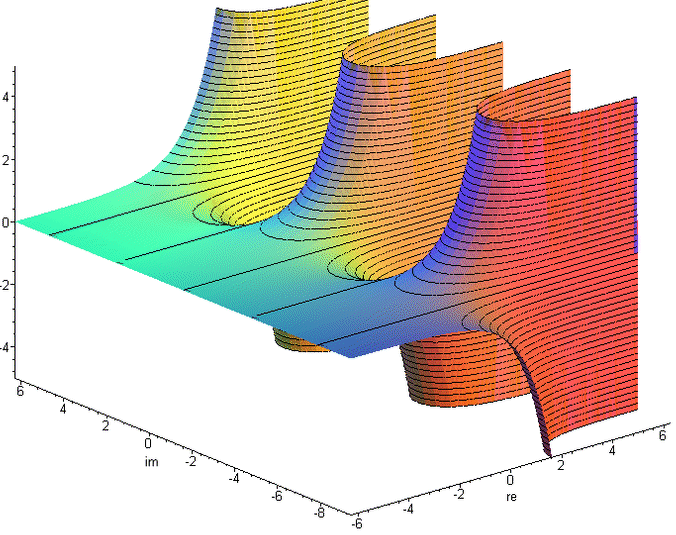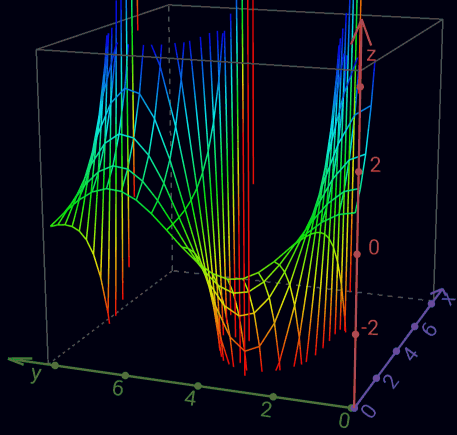Real part of the complex exponential function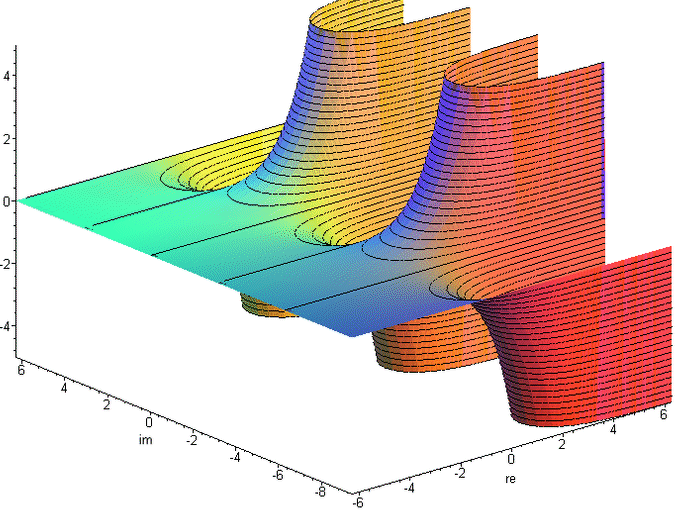Imaginary part of the complex exponential function

Transcendental
J  =  [<o>]                Phase Element            Definition   ln -1 = πi              (~3.1415926535i)
(J) = ([<o>]) = <o>         Involution2                           eJ = eπi = -1
(o)                         Euler number                          e1 = e                  (~2.71828182845)
((o))                                                             ee                      (~15.1542622414)
[<(a)>] = a[<o>]            Phase Independence of a  Theorem 7    ln -ea = a + ln -1
(a  JJ ) = (a)              J-cancellation1          Theorem 8    ea + 2*ln -1 = ea + ln (-1)² = ea + ln 1 = ea = ea + 2πi
(a<bJJ>) = (a<b>)           J-cancellation2          Theorem 9    ea - b - 2*ln -1 = ea - b - ln (-1)² = ea - b - ln 1 = ea - b = ea - b - 2πi
(J) = (<J>)                 Self-Inversion of J      Theorem 10   eJ = e-J
(([J]<[oo]>))               i and <i>                             eJ/2 =  √-1 = ±i
i = (<[<i>]>) = <(<[i]>)>   imaginary unit           Theorem 11   i = 1/-i = -e-ln i
([<J>][i]) = ([J][<i>])     Circle number Pi                      -J*i = J/i = J*-i = π   (~3.1415926535)

Like ln in the imaginary, by an arbitrary multiple of 2π is ambiguous, so at e all values are repeated every 2πi.
To represent this, the J-cancellation and self-inversion of J is permissible only within an instantiation,
or they must be contained in at least one more instantiation than in abstractions.

Proof of Phase Independence of a     for a != [∎]
[<(a)>              ]
[<(a)>( [         ])]  -Involution2
[<(a)>(a[         ])]  -Absorption of a
[<(a)>(a[o     <o>])]  -Inversion of o
[<(a)>(a[o])(a[<o>])]  Distribution of a
[<(a)>(a   )(a[<o>])]  Involution1
[           (a[<o>])]  Inversion of (a)
a[<o>]    Involution1

Proof of J-cancellation     within ()
[<o>][<o>]
[<([<o>])>]   -Phase Independence von [<o>]
[<  <o>  >]   Involution2
[    o    ]   Inverse Cancellation
Involution1

Proof of J-cancellation1     within ()
(a[<o>][<o>])      -Involution1
<<(a[ o ][ o ])>>    2x Inverse Promotion1
(a[ o ][ o ])      Inverse Cancellation
(a          )      2x Involution1

Proof of Self-Inversion of J     within ()
(J      )
(J<    >)   -Inversion of void
(J<J  J>)   -J-cancellation2
(J<J><J>)   -Inverse Collection
(    <J>)   Inversion of J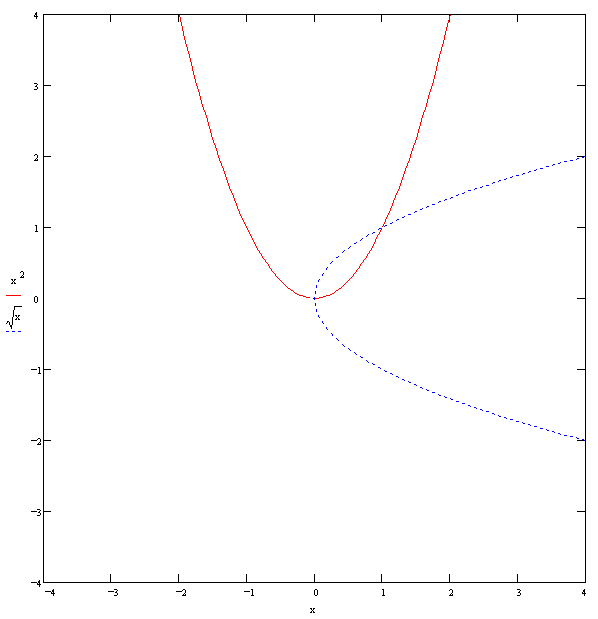f1(x) = x2
f2(x) = √x
f1(f2(x)) = x   (√x)2 = x
f2(f1(x)) = ±x   √(x2) = ±xReal part and imaginary part of the complex square root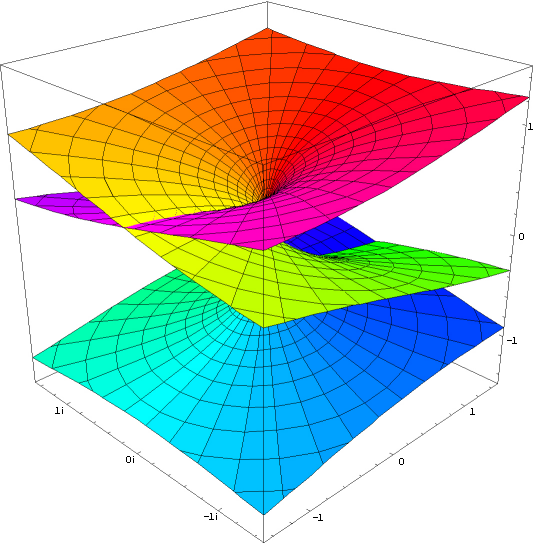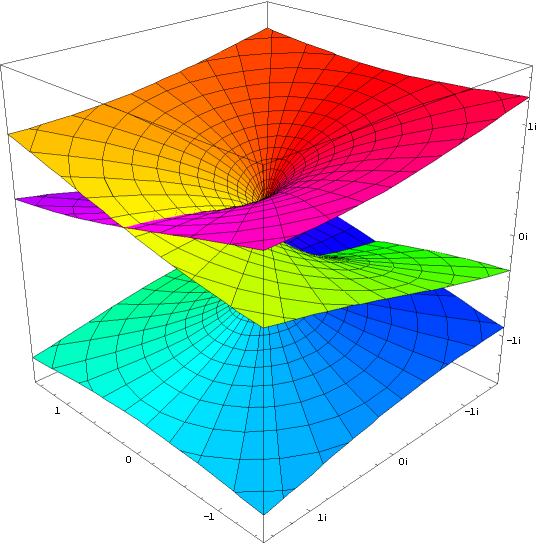Real part and imaginary part of the complex cube root

Proof of ambiguity of (([J]<[oo]>)) or √-1
Whenever the exponent is a non-integer rational number, there is ambiguity.
And if the exponent is less than or equal to 0, the base equals 0 gives a degenerate point.

i * i = -i * -i = (±i)2 = -1
([(([J]<[oo]>))][(([J]<[oo]>))])
(([[(([J]<[oo]>))]][oo]))       Cardinality of [(([J]<[oo]>))]
((    [J]<[oo]>    [oo]))       2x Involution1
((    [J]              ))       Inversion of [oo]
<o>                       2x Involution2
and
([<(([J]<[oo]>))>][<(([J]<[oo]>))>])
<<([ (([J]<[oo]>)) ][ (([J]<[oo]>)) ])>>   2x Inverse Promotion1
([ (([J]<[oo]>)) ][ (([J]<[oo]>)) ])     Inverse Cancellation
(([[(([J]<[oo]>))]][oo])           )     Cardinality of [(([J]<[oo]>))]
((    [J]<[oo]>    [oo])           )     2x Involution1
((    [J]              )           )     Inversion of [oo]
<o>                                2x Involution2

Proof of imaginary unit1
(<[<<(([ J ]<[oo]>))>>]>)
(<    ([ J ]<[oo]>)    >)   Inverse Cancellation, Involution1
(     ([<J>]<[oo]>)     )   -Inverse Promotion1
(     ([ J ]<[oo]>)     )   -Self-Inversion of J

Proof of imaginary unit2
<(<[(([ J ]<[oo]>))]>)>
<(<  ([ J ]<[oo]>)  >)>   Involution1
<(   ([<J>]<[oo]>)   )>   -Inverse Promotion1
<(   ([ J ]<[oo]>)   )>   -Self-Inversion of J

-1 * -1 = 1
([<o>][<o>])
(          )   J-cancellation1

ee = ee
(([[(o)]][(o)]))
((         o  ))   3x Involution1

<  a  >  = ([<([a])>]) = ([a][<o>]) = ([a]J)   2x-Involution2, Phase Independence of [a]
[<  a  >] =  [<([a])>]  =  [a][<o>]  =  [a]J    -Involution2, Phase Independence of [a]
<( a )>  = ([<( a )>]) =   (a[<o>]) =   (aJ)   -Involution2, Phase Independence von  a

Indefiniteness of Cardinality of J     within ()
... = (<JJJJ>) = (<JJ>) =  o  = (JJ) = (JJJJ) = ...     J-cancellation1, J-cancellation2, Inversion of void
... = (<JJJ>) = (<J>) = (J) = (JJJ) = (JJJJJ) = ...   J-cancellation1, J-cancellation2, Self-Inversion of J

Indefiniteness of [∎]     within ()
(   [∎]    )
(   [∎]  JJ)   -J-cancellation1
([<([∎])>]J)   -Phase Independence of [∎]
([<  ∎  >]J)   Involution2 - Indefiniteness der Inversion of ∎

Indefiniteness of 00
(([∎]∎))
((   ∎))   Absorption of [∎]
(      )   Involution2
=> 00 = 1 but from the Indefiniteness of [∎] follows in principle the Indefiniteness of 00

a0 = 1
(([[a]]∎))
((   ∎))   Absorption of [[a]]
(      )   Involution2

0n = 0   n ∈ ℕ
(([∎][o...o]))
(∎...∎)   Cardinality of ∎
(∎    )   Absorption of ∎
Involution2

ln 0 + ln -1 = ln 0
∎J
[<(∎)>]   -Phase Independence of ∎
[<   >]   Involution2
[     ]   Inversion of void

Proof of multiplicative Self-Inversion of (([J]<[oo]>)) (like o)
1/±i = ∓i
(<[(([ J ]<[oo]>))]>)
(<  ([ J ]<[oo]>)  >)   Involution1
(   ([<J>]<[oo]>)   )   -Inverse Promotion1
(   ([ J ]<[oo]>)   )   -Self-Inversion of J
and
(<[<(([ J ]<[oo]>))>]>)
<(<[ (([ J ]<[oo]>)) ]>)>   -Inverse Promotion2
<(<   ([ J ]<[oo]>)   >)>   Involution1
<(    ([<J>]<[oo]>)    )>   -Inverse Promotion1
<(    ([ J ]<[oo]>)    )>   -Self-Inversion of J

∓i = ±i
<(([ J ]<[oo]>))>
(<[<<(([ J ]<[oo]>))>>]>)   imaginary unit11
(<    ([ J ]<[oo]>)    >)   Inverse Cancellation, Involution1
(     ([<J>]<[oo]>)     )   -Inverse Promotion1
(     ([ J ]<[oo]>)     )   -Self-Inversion of J

±i = ∓i
(([ J ]<[oo]>))
<(<[(([ J ]<[oo]>))]>)>   imaginary unit12
<(<  ([ J ]<[oo]>)  >)>   Involution1
<(   ([<J>]<[oo]>)   )>   -Inverse Promotion1
<(   ([ J ]<[oo]>)   )>   -Self-Inversion of J

J/i = i*π / i = π
([J]<[(([ J ]<[oo]>))]>)
([J]<  ([ J ]<[oo]>)  >)   Involution1
([J]   ([<J>]<[oo]>)   )   -Inverse Promotion1
([J]   ([ J ]<[oo]>)   )   -Self-Inversion of J
and
([ J ]<[<(([ J ]<[oo]>))>]>)
<([ J ]<[ (([ J ]<[oo]>)) ]>)>   Inverse Promotion3
([<J>]<[ (([ J ]<[oo]>)) ]>)    -Inverse Promotion1
([<J>]<   ([ J ]<[oo]>)   >)    Involution1
([<J>]    ([<J>]<[oo]>)    )    -Inverse Promotion1
([<J>]    ([ J ]<[oo]>)    )    -Self-Inversion of J

J * -i = π
([ J ][<(([ J ]<[oo]>))>])
<([ J ][ (([ J ]<[oo]>)) ])>   Inverse Promotion1
([<J>][ (([ J ]<[oo]>)) ])    -Inverse Promotion1
([<J>]   ([ J ]<[oo]>)   )    Involution1
and
([J][<<(([ J ]<[oo]>))>>])
([J][  (([ J ]<[oo]>))  ])    Inverse Cancellation
([J]    ([ J ]<[oo]>)    )    Involution1

π * i = J
([([<J>][i])][i])
(  [<J>][i]  [i])     Involution1
(  [<J>][<o>   ])     i * i = -1
<<(  [ J ][ o    ])>>   2x Inverse Promotion1
J                Inverse Cancellation, Involution1, 2x Involution2

ii = eJ*i/2 = e-π/2 = e-π/2-2πk  k ∈ ℤ
(([[(([J]<[oo]>))]][(([J]<[oo]>))]))
((    [J]<[oo]>      ([J]<[oo]>)  ))   3x Involution1
and
( ([[(([ J ]<[oo]>))]][<(([J]<[oo]>))>]) )
(<(    [ J ]<[oo]>    [ (([J]<[oo]>)) ])>)   2x Involution1, Inverse Promotion1
( (    [<J>]<[oo]>    [ (([J]<[oo]>)) ]) )   -Inverse Promotion1
( (    [ J ]<[oo]>       ([J]<[oo]>)   ) )   Involution1, -Self-Inversion of J

i-2i = eπ
(([[(([J]<[oo]>))]][(([J]<[oo]>))][oo]))
((    [J]<[oo]>      ([J]<[oo]>)  [oo]))   3x Involution1
((    [J]            ([J]<[oo]>)      ))   Inversion of [oo]
and
( ([[(([ J ]<[oo]>))]][<(([J]<[oo]>))>][oo]) )
(<(    [ J ]<[oo]>    [ (([J]<[oo]>)) ][oo])>)   2x Involution1, Inverse Promotion1
( (    [<J>]<[oo]>    [ (([J]<[oo]>)) ][oo]) )   -Inverse Promotion1
( (    [ J ]             ([J]<[oo]>)       ) )   Involution1, -Self-Inversion of J

1/√a = √(1/a)
(<[(([ [a] ]<[oo]>))]>)
(<  ([ [a] ]<[oo]>)  >)   Involution1
(   ([<[a]>]<[oo]>)   )   -Inverse Promotion1

√9 = √±32 = ±3
(([[   ooooooooo   ]]<[oo]>))
(([[(  [ooo][ooo] )]]<[oo]>))   Cardinality of ooo
(([[(([[ooo]][oo]))]]<[oo]>))   Cardinality of [ooo]
((    [[ooo]][oo]    <[oo]>))   2x Involution1
((    [[ooo]]              ))   Inversion of [oo]
ooo                     2x Involution2
and
(([[       ooooooooo      ]]<[oo]>))
(([[  (  [ ooo ][ ooo ])  ]]<[oo]>))   Cardinality of ooo
(([[<<(  [ ooo ][ ooo ])>>]]<[oo]>))   -Inverse Cancellation
(([[  (  [<ooo>][<ooo>])  ]]<[oo]>))   2x -Inverse Promotion1
(([[  (([[<ooo>]][oo]) )  ]]<[oo]>))   Cardinality of [<ooo>]
((      [[<ooo>]][oo]       <[oo]>))   2x Involution1
((      [[<ooo>]]                 ))   Inversion of [oo]
<ooo>                        2x Involution2

-22 = 22
(([[<oo>]][oo]))
([<oo>][<oo>])     -Cardinality of [<oo>]
<<([ oo ][ oo ])>>   2x Inverse Promotion1
([ oo ][ oo ])     Inverse Cancellation
(([[oo]][oo]))       Cardinality of [oo]

ab = -ab
((      [[ a ]]                 [b]))
((      [[ a ]][oo]       <[oo]>[b]))   -Inversion of [oo]
(([[  (([[ a ]][oo]))  ] ]<[oo]>[b]))   2x -Involution1
(([[  (  [ a ][ a ] )  ] ]<[oo]>[b]))   -Cardinality of [a]
(([[<<(  [ a ][ a ] )>>] ]<[oo]>[b]))   -Inverse Cancellation
(([[  (  [<a>][<a>] )  ] ]<[oo]>[b]))   2x -Inverse Promotion
(([[  (([[<a>]][oo]))  ] ]<[oo]>[b]))   Cardinality of [<a>]
((      [[<a>]][oo]       <[oo]>[b]))   2x Involution1                      !!! ambiguity
((      [[<a>]]                 [b]))   Inversion of [oo]

Self-Inversion   problem
-0 = 0
1/1 = 1
<∎>
∎ = ∎∎ = ∎∎∎ = ∎∎∎∎ = ...
J = <J>            ... = <JJJJ> = <JJ> =   = JJ = JJJJ = ... , ambiguity of (([J]<[oo]>))
1/±i = ∓i
ab = -abThree-dimensional representation of the Eulerian formula ez*i = cos z + i sin z   =>  (([z]([J]<[oo]>)))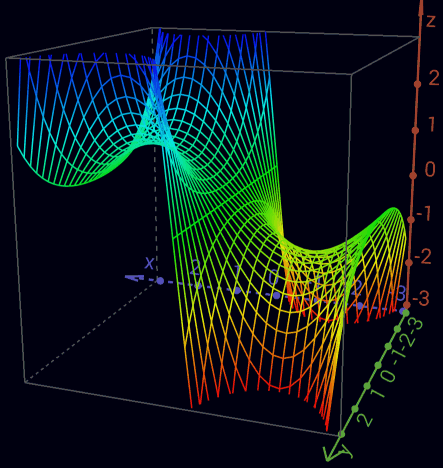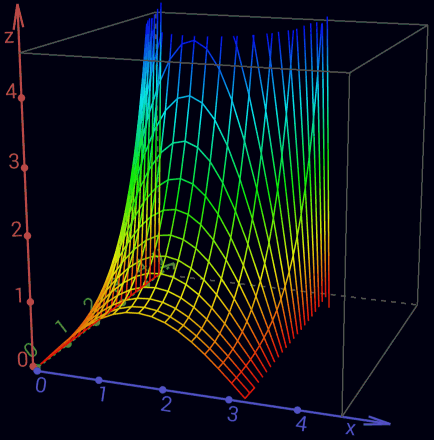Real part of the complex sine function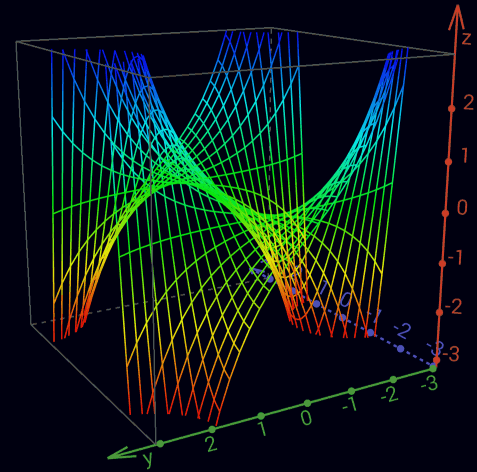Imaginary part of the complex sine function

an+1 =  ([an]J)      o -> (J) -> o -> (J) -> o -> ...  <=  (1 -> -1 ->  1 -> -1 -> 1 -> ...)
an+1 =  ([an][i])    o -> i -> (J) -> <i> -> o -> ...  <=  (1 ->  i -> -1 -> -i -> 1 -> ...)
an+1 = <([an][i])>   o -> <i> -> (J) -> i -> o -> ...  <=  (1 -> -i -> -1 ->  i -> 1 -> ...)

ea*i = cos a + i sin a    =>     (([a][i]))
e-a*i = cos a - i sin a   =>                 (([<a>][i]))
sin a = (ea*i - e-a*i)/2i  =>  ([ (([a][i]))<(([<a>][i]))>]<[oo][i]>)
cos a = (ea*i + e-a*i)/2   =>  ([ (([a][i])) (([<a>][i])) ]<[oo]   >)

ea  = cosh a + sinh a   =>     (a)
e-a = cosh a - sinh a   =>         (<a>)
sinh a = (ea - e-a)/2   =>   ([(a)<(<a>)>]<[oo]>)
cosh a = (ea + e-a)/2   =>   ([(a) (<a>) ]<[oo]>)

sin(x + i y) = sin(x) cosh(y) + i cos(x) sinh(y)
cos(x + i y) = cos(x) cosh(y) - i sin(x) sinh(y)
sin(z) = -i sinh(i z)
sinh(z) =  -i sin(i z)
cos(z) =    cosh(i z)
cosh(z) =     cos(i z)
sin'(z) =  cos(z)
sinh'(z) = cosh(z)
cos'(z) = -sin(z)
cosh'(z) = sinh(z)

unit circle    x2 + y2 = 1   (([[x]][oo])) (([[y]][oo]))  = o
unit hyperbole x2 - y2 = 1   (([[x]][oo]))<(([[y]][oo]))> = o

```